Thermodynamics and Propulsion

# 16.4 Thermal Resistance Circuits

There is an electrical analogy with conduction heat transfer that can be exploited in problem solving. The analog of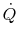is current, and the analog of the temperature difference,, is voltage difference. From this perspective the slab is a pure resistance to heat transfer and we can define(16..21)

where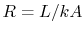, the thermal resistance. The thermal resistanceincreases asincreases, as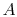decreases, and asdecreases.The concept of a thermal resistance circuit allows ready analysis of problems such as a composite slab (composite planar heat transfer surface). In the composite slab shown in Figure 16.6, the heat flux is constant with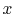. The resistances are in series and sum to. If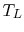is the temperature at the left, andis the temperature at the right, the heat transfer rate is given by(16..22)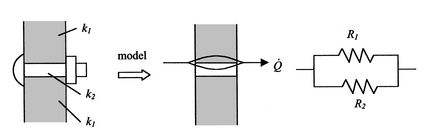Another example is a wall with a dissimilar material such as a bolt in an insulating layer. In this case, the heat transfer resistances are in parallel. Figure 16.7 shows the physical configuration, the heat transfer paths and the thermal resistance circuit.

For this situation, the total heat flowis made up of the heat flow in the two parallel paths,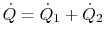, with the total resistance given by(16..23)

More complex configurations can also be examined; for example, a brick wall with insulation on both sides (Figure 16.8).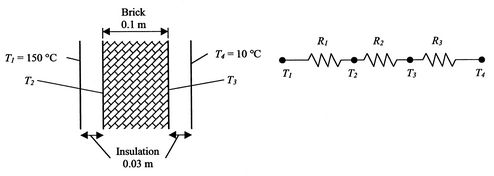The overall thermal resistance is given by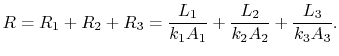(16..24)

Some representative values for the brick and insulation thermal conductivity are: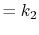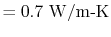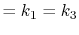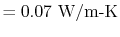Using these values, and noting that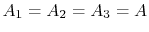, we obtain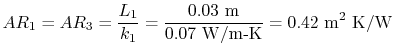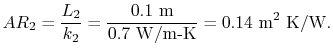This is a series circuit so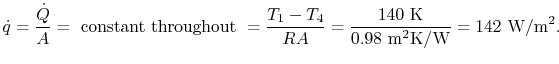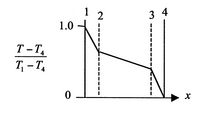The temperature is continuous in the wall and the intermediate temperatures can be found from applying the resistance equation across each slab, sinceis constant across the slab. For example, to find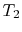: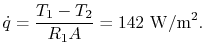This yields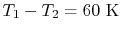or.

The same procedure gives. As sketched in Figure 16.9, the larger drop is across the insulating layer even though the brick layer is much thicker.

Muddy Points

What do you mean by continuous? (MP 16.4)

Why is temperature continuous in the composite wall problem? Why is it continuous at the interface between two materials? (MP 16.5)

Why is the temperature gradientnot continuous? (MP 16.6)

Why is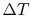the same for the two elements in a parallel thermal circuit? Doesn't the relative area of the bolt to the wood matter? (MP 16.7)

UnifiedTP